FEA assisted measurement of Young's modulus and Poisson's constant in stout horn blanks.

David Wuchinich

Modal Mechanics

Yonkers, NY

# Abstract

Measurement of the first flexural and the first torsional free-free resonant frequencies are employed to adjust assumed values for Young's modulus and Poisson's constant in finite element models until frequency equivalence is established.  The method yields, with simple production laboratory test equipment, measurement accuracies of one percent, thereby providing information necessary for precision computational assisted horn design.

# Introduction

Accurate design of extensional resonators whose lateral dimensions are comparable to their length, such as the horns used in plastic welding equipment, requires precise knowledge of both Young’s modulus and Poisson’s constant if substantial correction to their dimensions necessary to produce the desired resonant frequency is to be avoided.  Even for same alloy and specification, Young’s modulus may vary as much as ten percent from the nominal value given by the manufacturer, a variation that introduces a 5 percent variation in the horn frequency.  Most ultrasonic systems are intolerant of frequency deviations of more than 2 percent, a situation that often requires deliberate alteration of horn to achieve compliance.

The variation of Poisson’s constant within the same material type and specification is less known, but the constant, a measure of expected lateral motion in horns, plays an increasingly important role in determining frequency as the horns become stout.  The effect of coupled strains is always to lower the design frequency.  Again, to avoid post horn fabrication trimming, precise knowledge of this constant is necessary.

Typically, Young’s modulus and Poisson’s constant are measured in static strength testing fixtures where both the extension and lateral dilation are measured for known applied extensional strain.  This technique, while accurate, requires large and expensive hydraulic test stands and the preparation of specimens for which horn blanks having diameters comparable to their lengths are not be suitable candidates.

Finite element analysis (FEA) permits, given accurate material information, make-to-print horns that actually work as intended without post fabrication tuning.  It also offers a way to permit test frequency measurements on the horn blank itself, along with the blank dimensions and density, to be used to determine both the modulus and Poisson’s constant.

## Theory

Assuming a blank of uniform cross section and square faces, measurement may be made of both the first free-free flexural and torsional vibration frequencies, Ff and Ft respectively.  Both frequencies depend upon the square root of Young’s modulus, but the torsional frequency also depends directly upon Poisson’s constant, as the torsional sound velocity is proportional to the square root of the modulus of rigidity, G, also known as the shear modulus and defined as: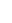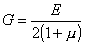(1)

where E is Young’s modulus and m denotes Poisson’s constant.  Ff, in the first approximation, depends only upon E.

To determine both constants, an FEA model is generated from the blank’s dimensions and the modal frequencies extracted from analysis using manufacturer’s data for the constants.  The model is constructed of meshes or regions whose further reduction in size does not change the computed frequencies within the precision needed.  The value of E is then corrected to produce in a second analysis (1st iteration) a value of Ff equal to that measured.

Attention is then directed to the computed value of Ft.  Using the expression given by Eq. 1 and noting that the torsional sound velocity varies as the square root of the quantity, correction is made to m to produce in a third analysis (2nd iteration) a computed value of Ft equal to that measured.

Returning the value of Ff produced by the 2nd iteration analysis, E is again corrected to produce in a fourth analysis (3rd iteration) equivalence between computation of Ff in computation and measurement.

The process continues focusing next upon Ft if necessary until sufficient precision is obtained in the result.  Because Ff is far less sensitive than Ft to changes in m, the computation will converge.  Figure 1 illustrates the computed relative frequency sensitivity of the extensional and torsional resonant frequencies to a fractional variation in Poisson’s constant and to the ratio of the length (L) to diameter (D) of a horn blank.  Figure 2 is a schematic representation of the iterative process.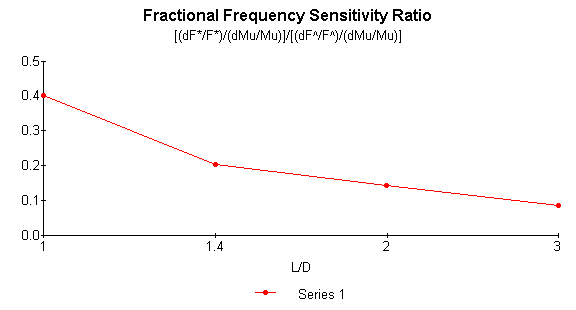Figure 1 – Computed Flexural/Torsional frequency sensitivity to Poisson's Constant in a round stout horn blank

 E1                                m1                                E2 Ffo, Fto   ®         Ff1= Ffm, Ft1        ®         Ff2, Ft2=Ftm         ®         Ff3=Ffm, Ft3 --®-- Ffn=Ffm, Ftn=Ftm                 1                                2                                3                             n Ffm, Ftm denote measured flexural and torsional frequencies

Figure 2 – Iterative computational procedure for determining Young’s modulus and Poisson’s constant.

In Figure 1 F* and F^ are the first free-free flexural and torsional frequencies respectively.  Mu denotes Poisson’s constant.  It is seen that, even when the length of blank equals its diameter, the flexural frequency is less than half as sensitive to changes in m than is the torsional frequency.

In place of Ff the extensional resonant frequency can also be used, but as, will be subsequently discussed, the flexural frequency can be obtained with simple test equipment and remains well defined at values of L/D near unity.

# Method

The apparatus used to measure the flexural resonance is sketched in Figure 3.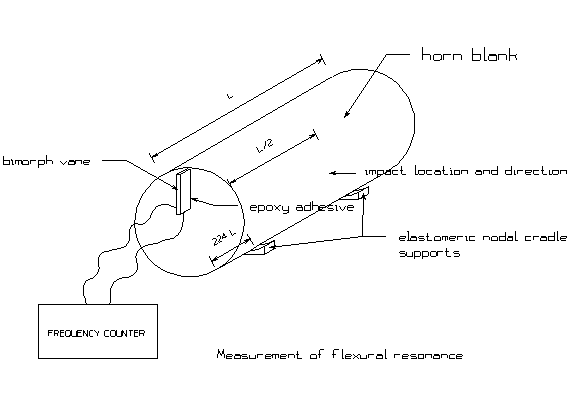Figure 3 - Measurement of Ff

The blank is supported by soft elastomeric supports at the location of the two nodes of the first free-free flexural mode, and the blank is struck with a ball peen hammer as shown at its center.  The flexural mode is easily excited in this manner.  A bimorph vane is cemented, using fast setting cyanoacrylate or epoxy adhesive, along a radius of one face with wire connections to its electrodes attached to a frequency meter.  The vane is positioned relative to the impact such that the resulting motion of the face is in a direction to flex the vane.  To ascertain that the frequency obtained is indeed the flexural vibration, a preliminary calculation can be done to determine the approximate expected value.  Even for blanks intended for subsequent life as 20 kHz horns whose diameter or largest lateral dimension equals the length, the first flexural frequency is usually audible.

Using the same blank and bimorph attachment, the measurement of the first free-free torsional mode is made as shown in Figure 4.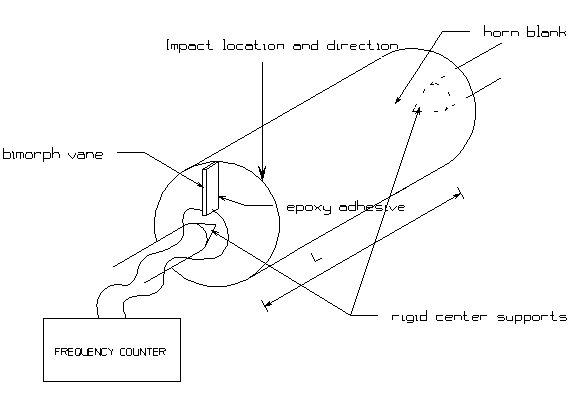Figure 4 - Measurement of Ft

The centers of the free faces are pinned, for example in lathe head and tail stock centers, to both support the blank and also suppress the excitation of extension and flexural modes.  Again, the approximate expected torsional frequency can be computed from the blank’s dimensions, given assumed values of the modulus and Poisson’s constant.  The blank is struck at one end asymmetrically to excite torsion.  As the modulus of rigidity is usually significantly smaller than Young’s modulus, the torsional frequency is also audible for blanks cut for use in 20 kHz extensional resonators.

In making both measurements, but particularly when measuring Ft, oscilloscope monitoring of the bimorph signal was found helpful initially in identifying excitation of the desired mode, a condition apparent in the progressive decay of the vibration over an interval of several seconds during which time the frequency indication remained the same.

# Results and Discussion

Two 6Al-4V titanium horn blanks were evaluated in this study.  Their dimensions, frequencies and computed values of frequencies, E and m are shown in Table 1.  Three iterations were required to bring the computed frequencies, Ffc and Ftc, within 0.2 percent of those measured.  Subsequent use of these blanks in horn designs produced operating frequencies within one percent of the calculation.

Blank

Diameter

mm

Length

mm

Ftm

Ftc

### Hz

Ffm

Hz

Ffc

Hz

#### E

GPa

m

1

63.6

134.4

11679

11679

11144

11127

114

0.288

2

82.5

141.2

11088

11064

11720

11716

112

0.288

Table 1

FEA assisted computation of material constants.

 The author acknowledges the gracious assistance of the Piezo-electric division of the Morgan Matroc Company in providing this PZT5H material, measuring approximately 6x25x0.5 mm.

 Kinsler, L and A. Frey, Fundamentals of Acoustics, 2nd Ed., John Wiley, p. 76 (1962).  To the first approximation, the flexural resonant frequency is determined by Young’s modulus, density, the radius of gyration of specimen cross section about the neutral axis and the square of the specimen length.

 Graff, K., Wave Motion in Elastic Solids, Dover Pub., pp. 125-127 (1991).  The torsional resonant frequency is determined by torsional rigidity (proportional to G), density, the polar moment of inertia of the specimen cross section and the specimen length.